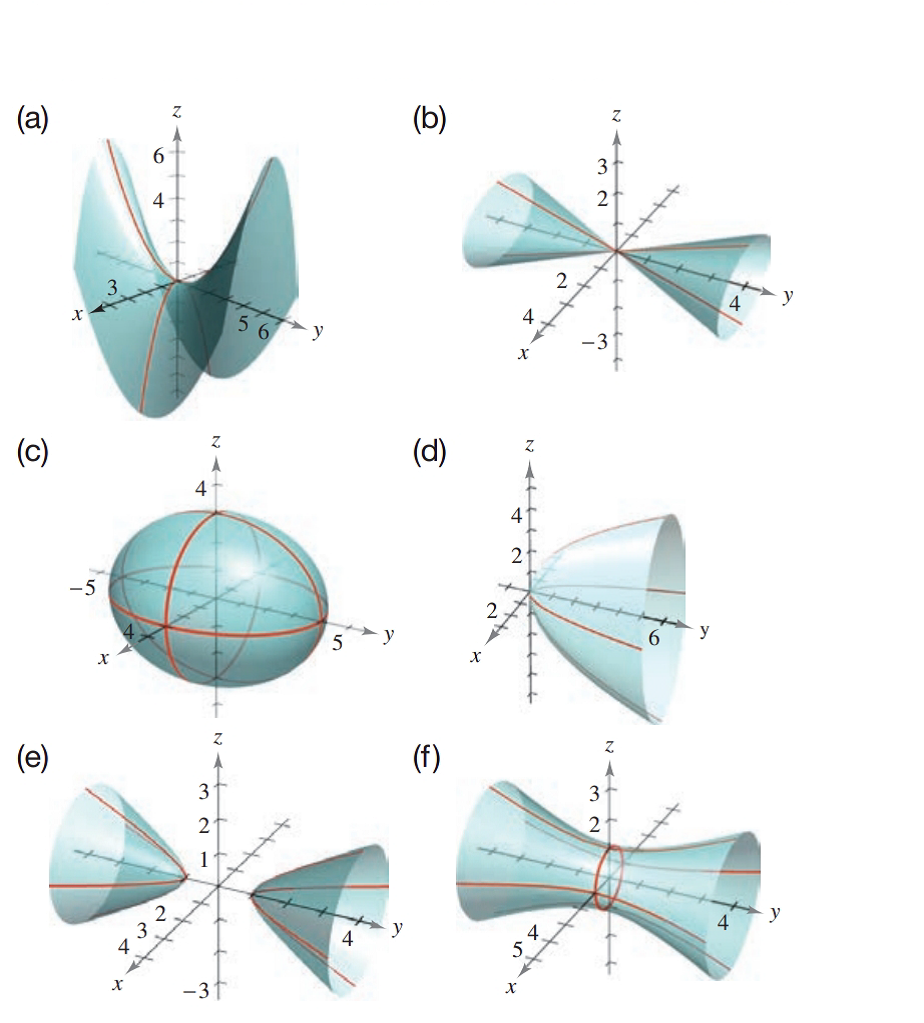Chapter 7.2, Problem 24E### Calculus: An Applied Approach (Min...

10th Edition
Ron Larson
ISBN: 9781305860919

#### Solutions

Chapter
Section### Calculus: An Applied Approach (Min...

10th Edition
Ron Larson
ISBN: 9781305860919
Textbook Problem
1 views

# Matching In Exercises 23-28, match the equation with its graph. [The graphs are labeled (a)-(f).]15 x 2 − 4 y 2 + 15 z 2 = − 4

To determine

The quadratic surface represented by the equation 15x24y2+15z2=4 and its correct graph from the provided graph.

Explanation

Given Information:

The provided quadratic surface equation is 15x24y2+15z2=4 and the provided option for matching as follows:

Consider the provided equation 15x24y2+15z2=4

Rewrite the equation as in standard format,

15x24y2+15z2=415x244y24+15z24=4415x244y24+15z24=115x2415z24+y21=1

Now, further simplify,

x2(415)+z2(415)y21=1x

### Still sussing out bartleby?

Check out a sample textbook solution.

See a sample solution

#### The Solution to Your Study Problems

Bartleby provides explanations to thousands of textbook problems written by our experts, many with advanced degrees!

Get Started

#### In Exercises 2336, find the domain of the function. 29. f(x)=5x

Applied Calculus for the Managerial, Life, and Social Sciences: A Brief Approach

#### What graph has f′(2) > 0 and f″(2) < 0?

Study Guide for Stewart's Single Variable Calculus: Early Transcendentals, 8th

#### The radius of convergence of is: 1 3 ∞

Study Guide for Stewart's Multivariable Calculus, 8th